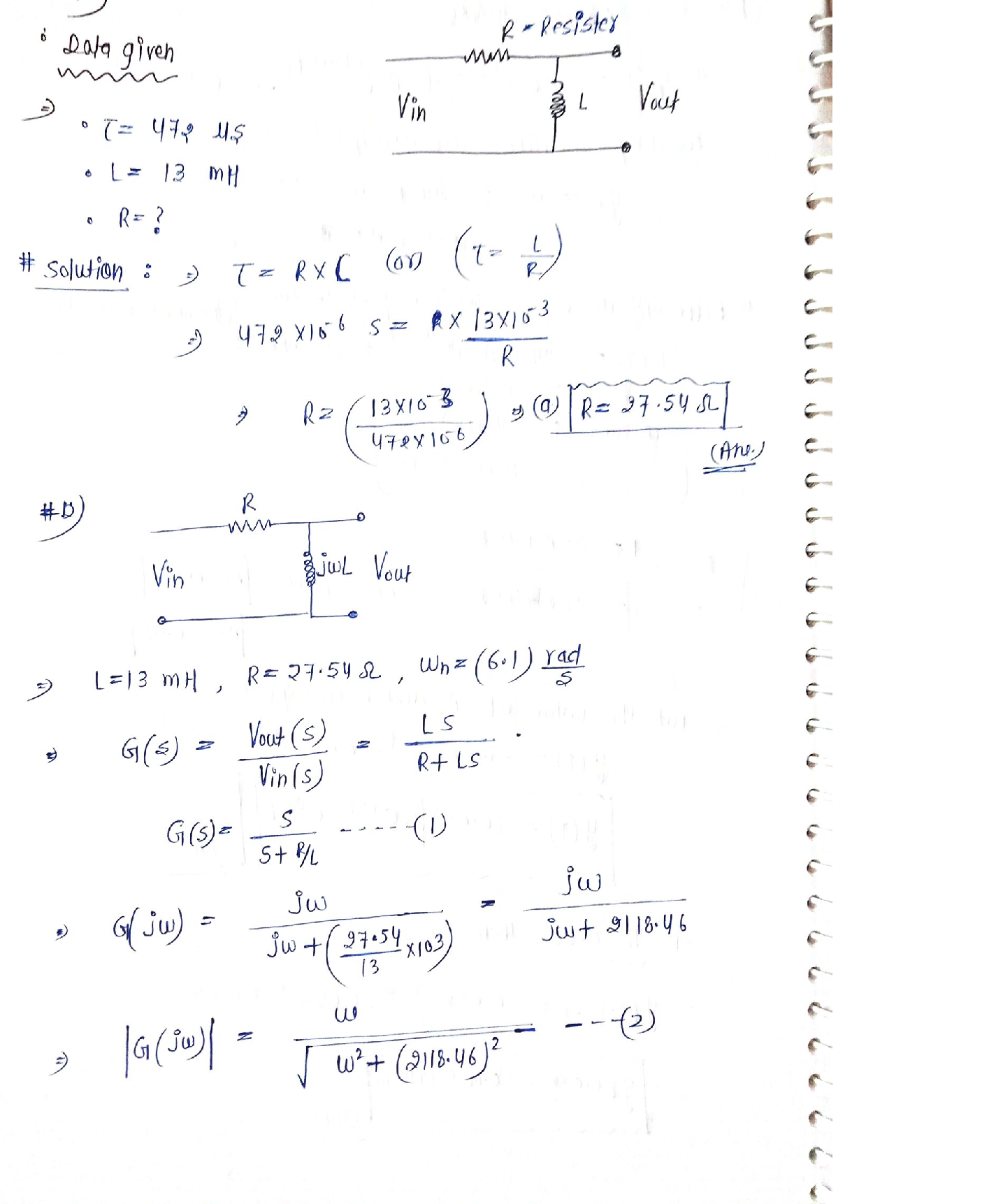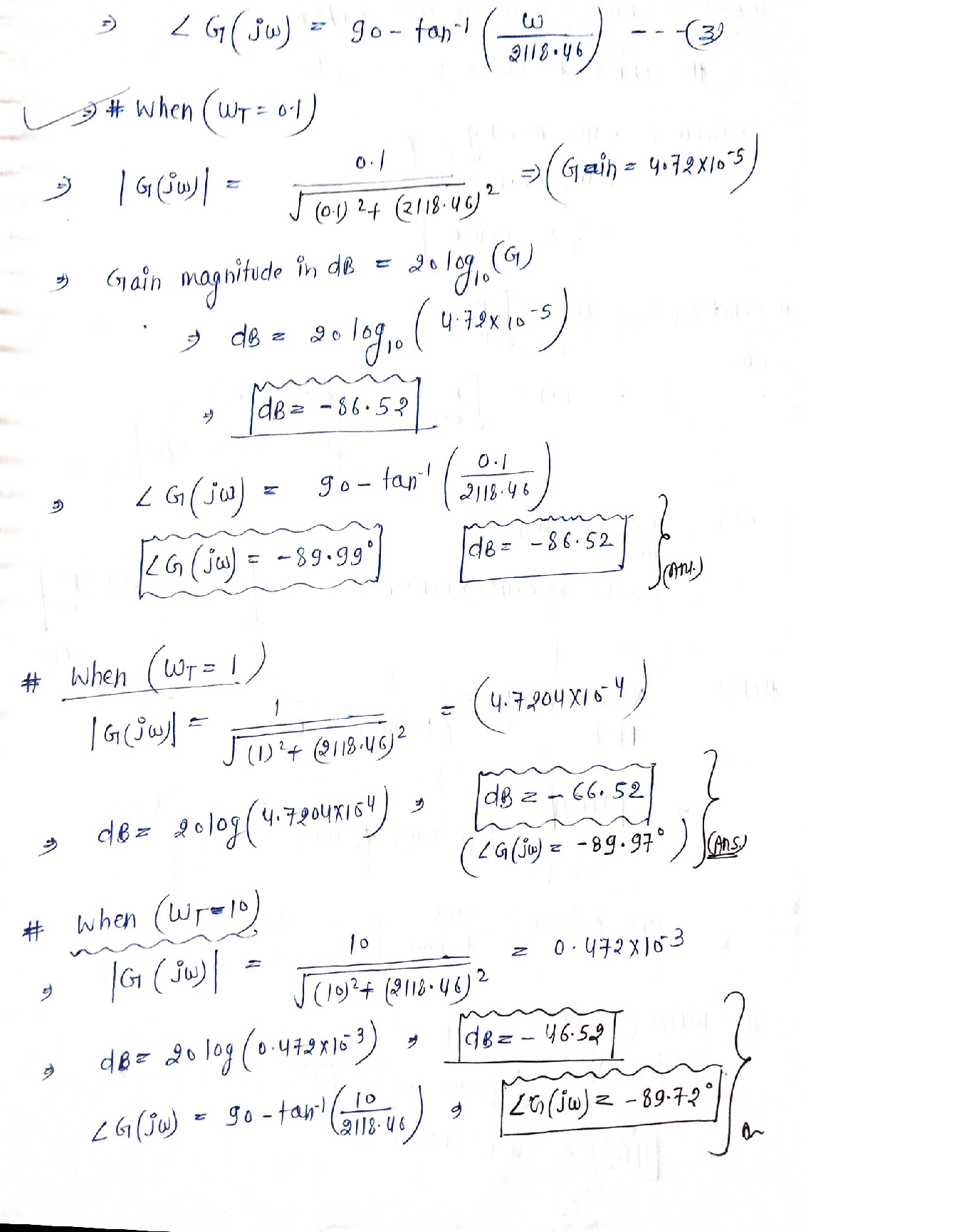Question

Solid Works

A passive, linear circuit consists of ideal resistor (R) and ideal inductor (L) in series. The system input is the total voltage (vVin) across both components; the output is defined as the voltage (vout) across the inductor. Calculate the:

(a) value of R (2) required to achieve the time constant t, which, together with L, is specified 5in the data sheet.

(b) Gain (dB) and phase (deg) of the transfer function vout/Vin at radian frequencies 0, such that OT = 0.1, 1.0, and 10.Verified### Question 45179Solid Works

An I-beam is made20 mm x 100 mm in cross section, nailed together with a single row of nails on top and bottom as shown.of three planks, each
If the longitudinal spacing between the nails is 25 mm,and the vertical shear force acting on the cross section is600 N, what is most nearly the shear per nail?

### Question 45178Solid Works

Problem 5: What is the bending stresses 0.05m above the neutral axis and is it tension or compression?

### Question 45177Solid Works

A wood beam (E=20 GPa, oall, wood=15 MPa) is reinforced with attaching steel plates (E=200 GPa, ol, Steel 160 MPa) on top and bottom of beam. Determine the maximum allowable bending moment M of this section.

### Question 45176Solid Works

Problem 3: Draw the shear and moment diagram for this beam and calculate the maximum bending stresses around the beam strong axis if the beam had rectangular cross section of 10inches deep and 3inches wide (25 points)

### Question 45175Solid Works

Problem 2: find the location of the centroid and moment of inertia about the Z-axis for this cross section(15 points)

### Question 45174Solid Works

Problem 1: Derive the maximum shear and moment value or equation for each of these beams in terms of w and P respectively (10 points) Hint: draw the shear and moment diagrams

### Question 38545Solid Works

If the allowable shear stress for each of the 10mm diameter pins at A, B and C is 90 MPa and the allowable normal stress for the 13 mm diameter rod BC is 150 MPa . Determine the largest intensity w of the uniform distributed load that can be suspended from the beam shown in the figure.

### Question 38544Solid Works

The assembly is used to support the distributed load w = 10 KN/m. Determine the factor of safety with respect to yielding for the steel rod BC and the pins at A and B if the yield stress for the steel is 250 MPa and in shear is 125 MPa. The rod has a diameter of 13 mm and the pins each have diameter of 10 mm. Furthermore, determine the amount of elongation in the rod BC knowing that the modulus of Elasticity of steel (E = 200 GPa).
Note: pins at A & C are subjected to double shear.

### Question 36263Solid Works

The slider-crank mechanism in Fig. Q4 is in equilibrium under the action of a vertical force P acting at A and a horizontal force F acting at B. Using the principle of virtual work or otherwise, determine the ratio F/P. The angle a and the ratio of lengths AB/OA (n) are specified in the data sheet.

### Question 36262Solid Works

A forced mass-spring-damper system obeys the following linear differential equation
m \ddot{x}+c \dot{x}+k x=f
The dependent variable 'x' represents displacement of the mass 'm'. The value of 'm', along with those of the specified undamped natural frequency on and damping ratio Ç are provided in the datasheet. Calculate the:
(a) Required values of c and k in Sl units
(b) Gain (dB) of the force-to-displacement transfer function x(s)/f(s) at angular (radian)frequencies o of 0.1, 1.0 and 10 times on.

### Submit query